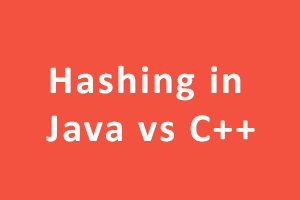Author - StudySection Post Views - 38 views# Hashing in Java vs C++

## Hashing

Hash tables, hash maps, and similar data structures allow indexing by a unique key, but that indexing is implemented in a very specific way. Hash tables essentially use an array, but that array is indexed by a hash value. Associative arrays are not supported by Java, however, using a Map, this could easily be resolved. C++ has std::unordered_map.

The idea with hashing is to convert a complex input value into a different value that can be used to extract or store data faster. Chain hashing collisions are avoided. The hash function is the value to link each cell of the hash table to a list of records.

Linear checking technique:- In this technique, each cell in a hash table has a single key-value pair. If a collision occurs by mapping a new key into the cell of the hash table, which is already occupied by another key. This method searches the table for the following nearest free space and inserts the new key there.

Examples:

### C++:

Inserting a key:-
``` Begin Insert(int a, int b) int hashVal = HashFunc(a) init = -1 deleteIndex = -1 while (hashVal != init and (ht[hashVal]==delNode::getNode() or ht[hashVal]!= NULL and ht[hashVal]->a != a)) if (init == -1) init = hashVal if (ht[hashVal] == delNode::getNode()) deleteIndex = hashVal hashVal = HashFunc(hashVal + 1) if (ht[hashVal] == NULL || hashVal == init) if(deleteIndex != -1) ht[deleteIndex] = new HashTable(a, b) else ht[hashVal] = new HashTable(a, b) if(init != hashVal) if (ht[hashVal] != delNode::getNode()) if (ht[hashVal] != NULL) if (ht[hashVal]->a== a) ht[hashVal]->b = b else ht[hashVal] = new HashTable(a, b) End```

``` (adsbygoogle = window.adsbygoogle || []).push({}); ```
``` ```

```Delete:- Begin Remove(int a) int hashVal = HashFunc(a) init = -1 while (hashVal != init and (ht[hashVal] == delNode::getNode() or ht[hashVal]!= NULL and ht[hashVal]->a!= a)) if (init == -1) init = hashVal hashVal = HashFunc(hashVal + 1) if (hashVal != init && ht[hashVal] != NULL) delete ht[hashVal] ht[hashVal] = delNode::getNode() End ```

### Java:

``` import java.util.*; class Test { public static void main(String[] args) { HashSet h = new HashSet(); ```

``` ```

``` // Adding elements into HashSet using add() h.add("element1"); h.add("element2"); h.add("element3"); h.add("element1");// adding duplicate elements System.out.println(h); // Checking if element1 is present or not System.out.println("\nHashSet contains element or not:" + h.contains("element1")); h.remove("element2"); System.out.println("\nList after removing element2:" + h); System.out.println("\nIterating over list:"); Iterator i = h.iterator(); while (i.hasNext()) System.out.println(i.next()); } } ```

Knowledge of .NET is quite rewarding in the IT industry. If you have got some skills in the .NET framework then a .NET certification from StudySection can prove to be a good attachment with your resume. You can go for a foundation level certificate as well as an advanced level certificate in the .NET framework.